This example shows how to: - Include survey weights in a multi-level model - Produce small area estimates of obesity prevalence in US cities using the BRFSS

The example merges data from the 2014 CDC Behavioral Risk Factor Surveillance System (BRFSS) SMART MSA data. Link and the 2010 American Community Survey 5-year estimates at the MSA level. More details on these data are found below.

#load brfss
library(car)
library(survey)
library(knitr)
library(lme4)
set.seed(12345)
samps<-sample(1:nrow(brfss14), size = 90000, replace=F)
#brfss14<-brfss14[samps,]
#The names in the data are very ugly, so I make them less ugly
nams<-names(brfss14)
#we see some names are lower case, some are upper and some have a little _ in the first position. This is a nightmare.
newnames<-gsub(pattern = "x_",replacement =  "",x =  nams)
names(brfss14)<-tolower(newnames)

#BMI
brfss14$bmi<-ifelse(is.na(brfss14$bmi5)==T, NA, brfss14$bmi5/100) #Poor or fair self rated health #brfss14$badhealth<-ifelse(brfss14$genhlth %in% c(4,5),1,0) brfss14$badhealth<-recode(brfss14$genhlth, recodes="4:5=1; 1:3=0; else=NA") #race/ethnicity brfss14$black<-recode(brfss14$racegr3, recodes="2=1; 9=NA; else=0") brfss14$white<-recode(brfss14$racegr3, recodes="1=1; 9=NA; else=0") brfss14$other<-recode(brfss14$racegr3, recodes="3:4=1; 9=NA; else=0") brfss14$hispanic<-recode(brfss14$racegr3, recodes="5=1; 9=NA; else=0") brfss14$race_eth<-recode(brfss14$racegr3, recodes="1='nhwhite'; 2='nh black'; 3:4='nh other';5='hispanic'; else=NA", as.factor.result = T) brfss14$race_eth<-relevel(brfss14$race_eth, ref = "nhwhite") #insurance brfss14$ins<-ifelse(brfss14$hlthpln1==1,1,0) #income grouping brfss14$inc<-ifelse(brfss14$incomg==9, NA, brfss14$incomg)

#education level
brfss14$educ<-recode(brfss14$educa, recodes="1:2='0Prim'; 3='1somehs'; 4='2hsgrad';
#brfss14$educ<-relevel(brfss14$educ, ref='0Prim')

#employment
brfss14$employ<-recode(brfss14$employ, recodes="1:2='Employed'; 2:6='nilf';
7='retired'; 8='unable'; else=NA", as.factor.result=T)
brfss14$employ<-relevel(brfss14$employ, ref='Employed')

#marital status
brfss14$marst<-recode(brfss14$marital, recodes="1='married'; 2='divorced'; 3='widowed';
4='separated'; 5='nm';6='cohab'; else=NA", as.factor.result=T)
brfss14$marst<-relevel(brfss14$marst, ref='married')

#Age cut into intervals
brfss14$agec<-cut(brfss14$age80, breaks=c(0,24,39,59,79,99), include.lowest = T)

## Standardizing Survey Weights

Here is an example of using weights that sum to the MSA specific sample size. I follow the first method described by Carle (2009).

First, I make an id that is the strata. Then I sum the weights within strata and count up the number of people in each strata.

Then I merge these back to the data, and standardize the weights

brfss14$sampleid<-brfss14$ststr
#within each strata, sum the weights
wts<-tapply(brfss14$mmsawt,brfss14$sampleid,sum)
#make a data frame from this
wts<-data.frame(id=names(unlist(wts)), wt=unlist(wts))
#get the unique sampling location ids'
t1<-as.data.frame(table(brfss14$sampleid)) #put all of this into a data set wts2<-data.frame(ids=wts$id, sumwt=wts$wt, jn=t1$Freq)
#merge all of this back to the original data file
brfss14<-merge(brfss14, wts2, by.x="sampleid", by.y="ids", all.x=T)
#In the new data set, multiply the original weight by the fraction of the
#sampling unit total population each person represents
brfss14$swts<-brfss14$mmsawt*(brfss14$jn/brfss14$sumwt)

#compare standardized weights to old person weights
hist(brfss14$swts); summary(brfss14$swts)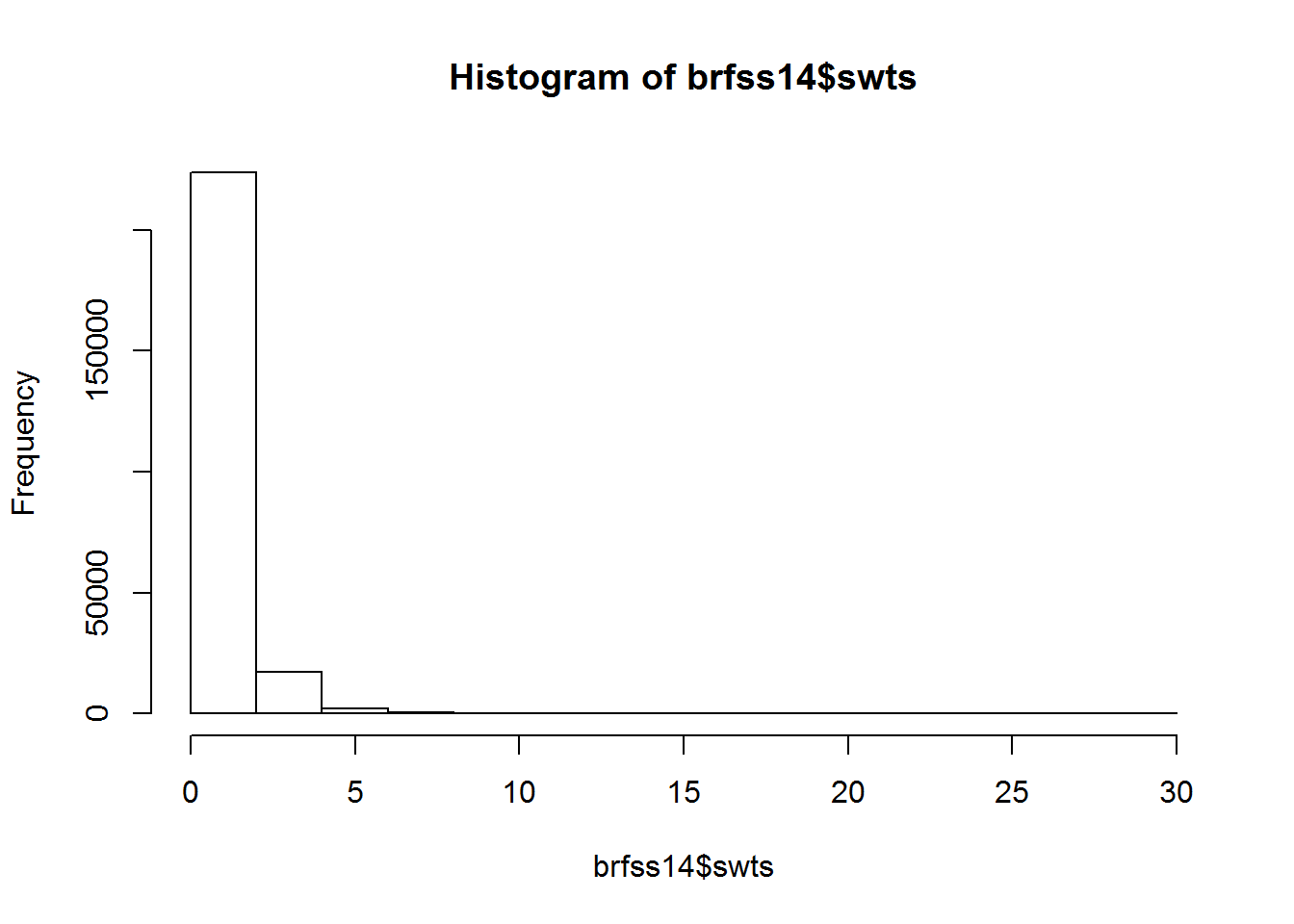##      Min.   1st Qu.    Median      Mean   3rd Qu.      Max.
##  0.007311  0.506700  0.791100  1.000000  1.229000 28.960000
hist(brfss14$mmsawt); summary(brfss14$mmsawt)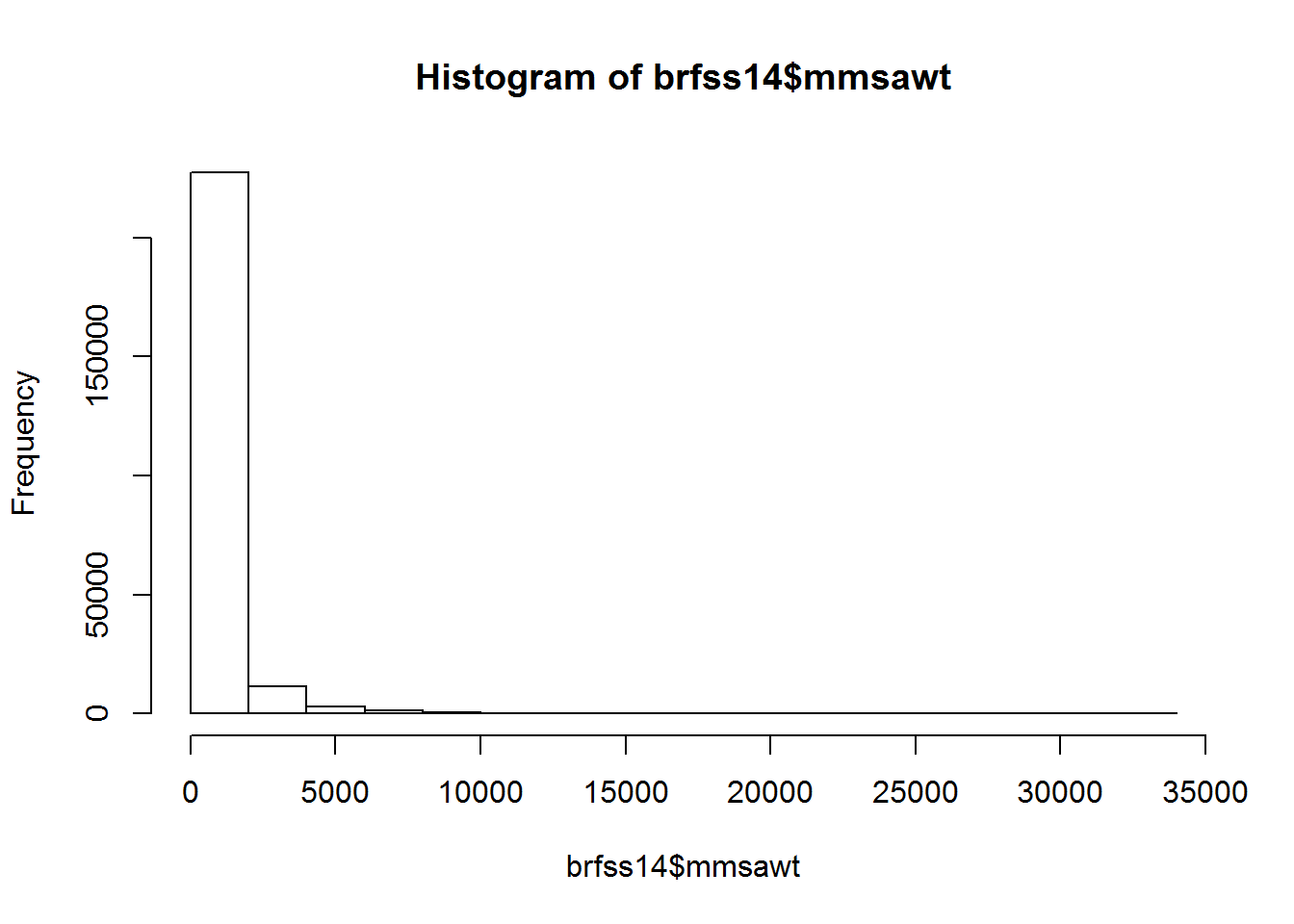##    Min. 1st Qu.  Median    Mean 3rd Qu.    Max.
##     1.3   113.4   263.3   619.8   640.8 32860.0

And we can see the effect of standardizing the weights.

library(acs)
#Get 2010 ACS median household incomes for tracts in Texas
msaacs<-geo.make(msa="*")

#ACS table B03002 is Hispanic ethnicity by race
acsecon<-acs.fetch(key=mykey, endyear=2010, span=5, geography=msaacs, variable = c("B19083_001","B17001_001","B17001_002", "B03002_001","B03002_004", "B03002_012" ))

colnames(acsecon@estimate)
##  "B19083_001" "B17001_001" "B17001_002" "B03002_001" "B03002_004"
##  "B03002_012"
msaecon<-data.frame(gini=acsecon@estimate[, "B19083_001"],
ppoverty=acsecon@estimate[, "B17001_002"]/acsecon@estimate[, "B17001_001"],
pblack=acsecon@estimate[,"B03002_004"]/acsecon@estimate[, "B03002_001"],
phisp=acsecon@estimate[,"B03002_012"]/acsecon@estimate[, "B03002_001"],
giniz=scale(acsecon@estimate[, "B19083_001"]),
ppovertyz=scale(acsecon@estimate[, "B17001_002"]/acsecon@estimate[, "B17001_001"]))
msaecon$zpblack<-scale(msaecon$pblack)
msaecon$zphisp<-scale(msaecon$phisp)
msaecon$ids<-paste(acsecon@geography$metropolitanstatisticalareamicropolitanstatisticalarea)

Let’s see the geographic variation in these economic indicators:

library(tigris)
##
## Attaching package: 'tigris'
## The following object is masked from 'package:graphics':
##
##     plot
msa<-core_based_statistical_areas(cb=T)
msa_ec<-geo_join(msa, msaecon, "CBSAFP", "ids", how="inner")

library(RColorBrewer)
library(sp)
spplot(msa_ec, "gini", at=quantile(msa_ec$gini), col.regions=brewer.pal(n=6, "Reds"), col="transparent", main="Gini Coefficient")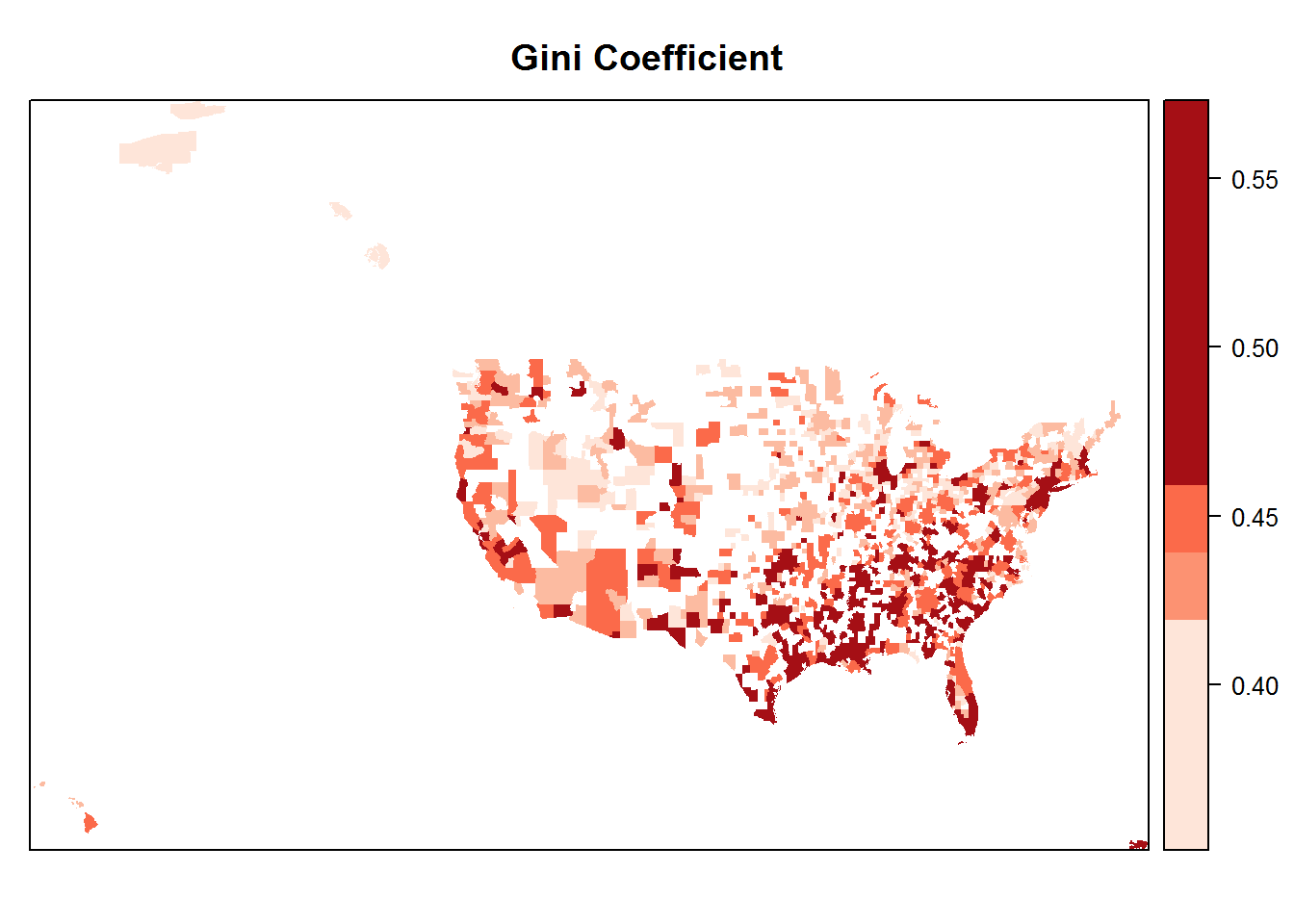spplot(msa_ec, "phisp", at=quantile(msa_ec$phisp), col.regions=brewer.pal(n=6, "Reds"), col="transparent", main="Percent Hispanic")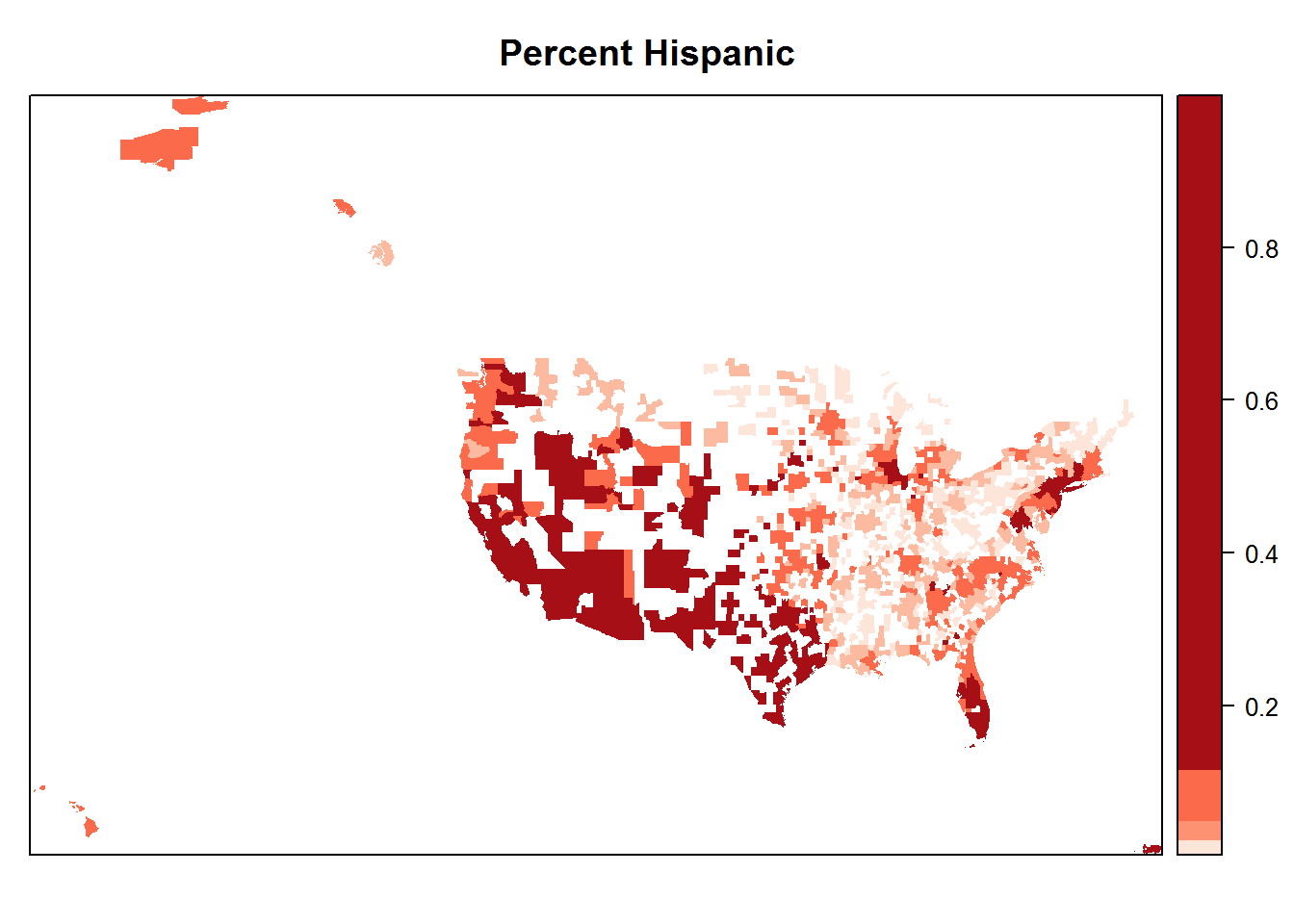Merge the MSA data to the BRFSS data

joindata<-merge(brfss14, msaecon, by.x="mmsa",by.y="ids", all.x=T)
joindata$bmiz<-scale(joindata$bmi, center=T, scale=T)
joindata<-joindata[complete.cases(joindata[, c("bmiz", "race_eth", "agec", "educ", "gini")]),]
#and merge the data back to the kids data

head(joindata[, c("bmiz", "race_eth", "agec", "educ","ppoverty", "pblack", "phisp", "mmsaname")])
##         bmiz race_eth    agec     educ  ppoverty      pblack      phisp
## 2  1.5160997  nhwhite (39,59] 3somecol 0.1034143 0.003095512 0.01500325
## 3  0.5923438  nhwhite (24,39] 4colgrad 0.1034143 0.003095512 0.01500325
## 4  0.8572200  nhwhite (24,39] 4colgrad 0.1034143 0.003095512 0.01500325
## 5 -0.4373626  nhwhite (39,59] 4colgrad 0.1034143 0.003095512 0.01500325
## 6  0.5393685  nhwhite (59,79] 3somecol 0.1034143 0.003095512 0.01500325
## 7  0.9482712  nhwhite (79,99]  2hsgrad 0.1034143 0.003095512 0.01500325
##                                      mmsaname
## 2 Aberdeen, SD, Micropolitan Statistical Area
## 3 Aberdeen, SD, Micropolitan Statistical Area
## 4 Aberdeen, SD, Micropolitan Statistical Area
## 5 Aberdeen, SD, Micropolitan Statistical Area
## 6 Aberdeen, SD, Micropolitan Statistical Area
## 7 Aberdeen, SD, Micropolitan Statistical Area

The glmer() function will estimate this model. First, I will estimate the model without survey weights, then again with survey weights that we standardized above.

fit.mix.bin<-glmer(I(bmi>30)~agec+educ+race_eth+(1|mmsa), family=binomial, joindata,
control=glmerControl(optimizer="bobyqa",optCtrl=list(maxfun=2e5)))
arm::display(fit.mix.bin, detail=T)
## glmer(formula = I(bmi > 30) ~ agec + educ + race_eth + (1 | mmsa),
##     data = joindata, family = binomial, control = glmerControl(optimizer = "bobyqa",
##         optCtrl = list(maxfun = 2e+05)))
##                  coef.est coef.se z value Pr(>|z|)
## (Intercept)       -1.57     0.05  -33.44    0.00
## agec(24,39]        0.81     0.03   26.10    0.00
## agec(39,59]        1.08     0.03   36.93    0.00
## agec(59,79]        0.97     0.03   33.19    0.00
## agec(79,99]        0.08     0.04    2.32    0.02
## educ1somehs        0.02     0.04    0.45    0.66
## educ2hsgrad       -0.10     0.04   -2.69    0.01
## educ3somecol      -0.12     0.04   -3.29    0.00
## educ4colgrad      -0.52     0.04  -14.53    0.00
## race_ethhispanic   0.19     0.02    8.26    0.00
## race_ethnh black   0.55     0.02   29.00    0.00
## race_ethnh other  -0.09     0.03   -3.21    0.00
##
## Error terms:
##  Groups   Name        Std.Dev.
##  mmsa     (Intercept) 0.14
##  Residual             1.00
## ---
## number of obs: 175811, groups: mmsa, 114
## AIC = 205209, DIC = 204612.4
## deviance = 204897.5
fit.mix.bin.wt<-glmer(I(bmi>30)~agec+educ+race_eth+(1|mmsa), family=binomial, joindata,
weights = swts,
control=glmerControl(optimizer="bobyqa",optCtrl=list(maxfun=2e5)))
## Warning: non-integer #successes in a binomial glm!
arm::display(fit.mix.bin.wt, detail=T)
## glmer(formula = I(bmi > 30) ~ agec + educ + race_eth + (1 | mmsa),
##     data = joindata, family = binomial, control = glmerControl(optimizer = "bobyqa",
##         optCtrl = list(maxfun = 2e+05)), weights = swts)
##                  coef.est coef.se z value Pr(>|z|)
## (Intercept)       -1.61     0.04  -41.28    0.00
## agec(24,39]        0.85     0.02   36.73    0.00
## agec(39,59]        1.10     0.02   50.08    0.00
## agec(59,79]        1.00     0.02   43.41    0.00
## agec(79,99]        0.14     0.04    3.86    0.00
## educ1somehs        0.02     0.03    0.49    0.62
## educ2hsgrad       -0.06     0.03   -2.10    0.04
## educ3somecol      -0.07     0.03   -2.38    0.02
## educ4colgrad      -0.53     0.03  -16.92    0.00
## race_ethhispanic   0.13     0.02    6.46    0.00
## race_ethnh black   0.50     0.02   26.69    0.00
## race_ethnh other  -0.24     0.03   -8.61    0.00
##
## Error terms:
##  Groups   Name        Std.Dev.
##  mmsa     (Intercept) 0.14
##  Residual             1.00
## ---
## number of obs: 175811, groups: mmsa, 114
## AIC = 188261, DIC = 221192.5
## deviance = 204713.5

Which in this case leads to interpretations of the results that are very similar between the models. The only parameter that differs much between the models is the nh other group, whose estimate is much larger in the model with weights included. This suggests that the weights are probably accounting for the un-representation of this demographic group in the data.

### Small area estimation

This example will illustrate a way to combine individual survey data with aggregate data on MSAs to produce a MSA level estimate of basically any health indicator measured using the BRFSS. The framework I use below takes observed individual level survey responses from the BRFSS and merges these to MSA level variables from the ACS. This allows me to estimate the overall regression model for MSA-level prevalence, controlling for MSA level variables. Then, I can use this equation for prediction for MSAs where I have not observed survey respondents, but for which I have observed the MSA level characteristics.

This corresponds to a multilevel logistic model with a higher level variables as predictors and can be written: $ln \frac {p_{ij}}{1-p{ij}} = \beta_{0j} + \sum {\gamma z_j}$

fit.msa<-glmer(I(bmi>30)~zpblack+zphisp+ppovertyz+(1|mmsa), family=binomial, joindata,
weights=swts,
control=glmerControl(optimizer="bobyqa",optCtrl=list(maxfun=2e5)))
## Warning: non-integer #successes in a binomial glm!
arm::display(fit.msa, detail=T)
## glmer(formula = I(bmi > 30) ~ zpblack + zphisp + ppovertyz +
##     (1 | mmsa), data = joindata, family = binomial, control = glmerControl(optimizer = "bobyqa",
##     optCtrl = list(maxfun = 2e+05)), weights = swts)
##             coef.est coef.se z value Pr(>|z|)
## (Intercept)  -0.83     0.02  -41.11    0.00
## zpblack       0.07     0.02    3.24    0.00
## zphisp       -0.03     0.03   -1.23    0.22
## ppovertyz     0.06     0.03    2.33    0.02
##
## Error terms:
##  Groups   Name        Std.Dev.
##  mmsa     (Intercept) 0.16
##  Residual             1.00
## ---
## number of obs: 175811, groups: mmsa, 114
## AIC = 193782, DIC = 227569.5
## deviance = 210670.8

Here I find predicted values for the counties with no individual data, but with observed county variables. In the brfss I have 132 MSAs, but according to the map data above, there are really 878 MSAs in the country. So, I can use my model to estimate the obesity prevalence for the other 746 MSAs that are not in the data.

To do this, I need to make a data set that has the observed MSA level variables for all MSAs, including the ones that aren’t in our our brfss data

#All msas, with ACS data attached
msas<-msa_ec
msas$mmsa<-msas$CBSAFP
msas$fitted<-predict(fit.msa, newdata=msas[, c("zpblack","zphisp","ppovertyz", "mmsa", "NAME")], allow.new.levels=T,type="response" ) head(msas@data[, c("NAME","zpblack","zphisp","ppovertyz", "fitted" )]) ## NAME zpblack zphisp ppovertyz ## 0 Sioux Falls, SD -0.5229221 -0.45819688 -1.1555786 ## 1 Augusta-Richmond County, GA-SC 1.9777653 -0.41383735 0.1766050 ## 2 Durham-Chapel Hill, NC 1.3251195 -0.05809925 -0.1080849 ## 3 Cleveland-Elyria, OH 0.7937601 -0.39053095 -0.3865475 ## 4 Monroe, LA 1.9430479 -0.52697550 0.6871122 ## 5 Fort Smith, AR-OK -0.4442094 -0.21013742 0.3675384 ## fitted ## 0 0.2828338 ## 1 0.3153970 ## 2 0.3221147 ## 3 0.3097104 ## 4 0.3461725 ## 5 0.3039697 #Map my estimates library(sp) library(RColorBrewer) spplot(msas, "fitted", at=quantile(msas$fitted, na.rm=T), col.regions=brewer.pal(n=5, "Blues"), main="My Estimates of %Obesity Prevalence in MSAs using BRFSS")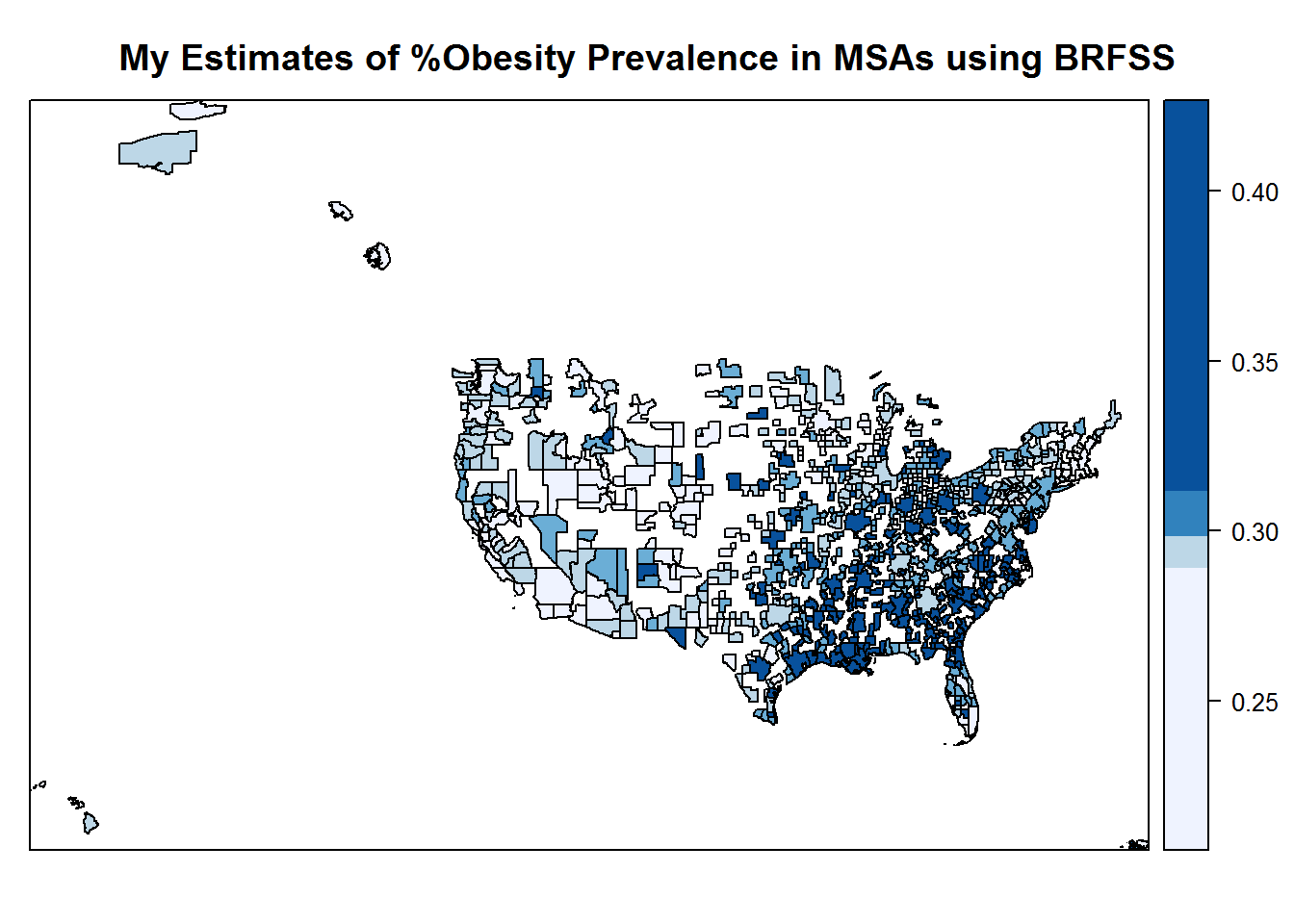For an example, here is our estimate of the % obese from the model, for San Antonio, TX.

msas@data[grep(pattern = "San Antonio", msas\$NAME),c("NAME","zpblack","zphisp","ppovertyz", "fitted" )]
##                              NAME    zpblack   zphisp   ppovertyz
## 155 San Antonio-New Braunfels, TX -0.2449772 2.329703 -0.08529324
##        fitted
## 155 0.3131013

while this report puts the rate in 2012 at 29%, and those data are based on a special BRFSS for Bexar county, conducted by the county health department.

In this type of situation, one should try to find a way to “check” to see how bad your estimates are, but in this case, I cannot find another source for MSA level obesity prevalences. In a previous semester, I did this analysis for US counties and compared those results to US county obesity prevalences from the CDC from here.